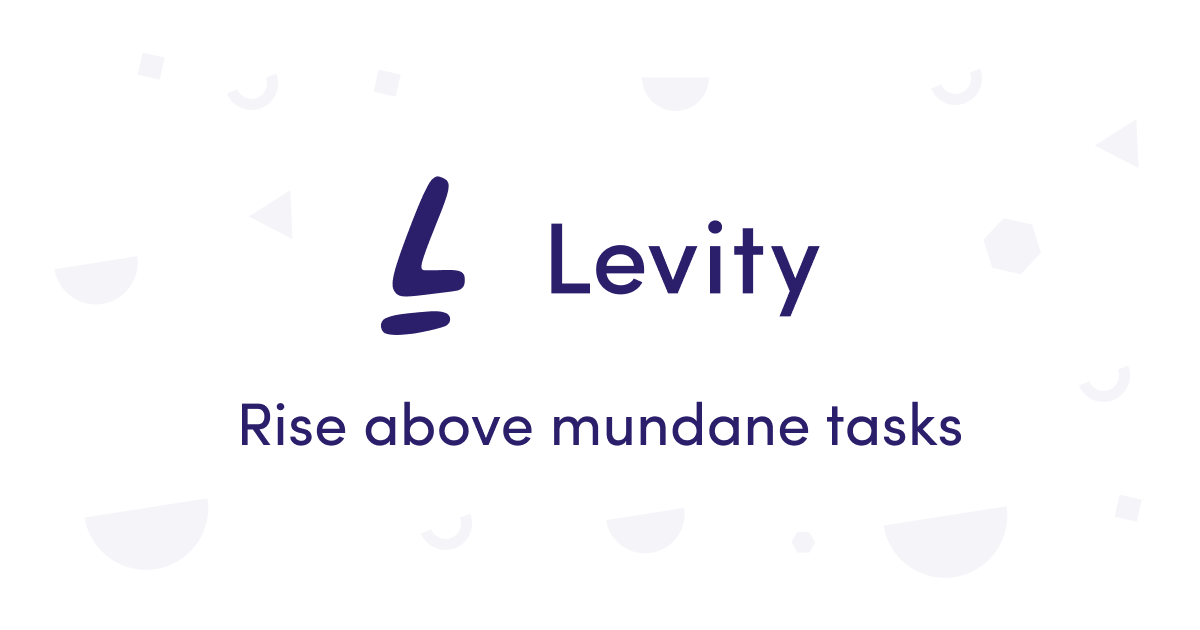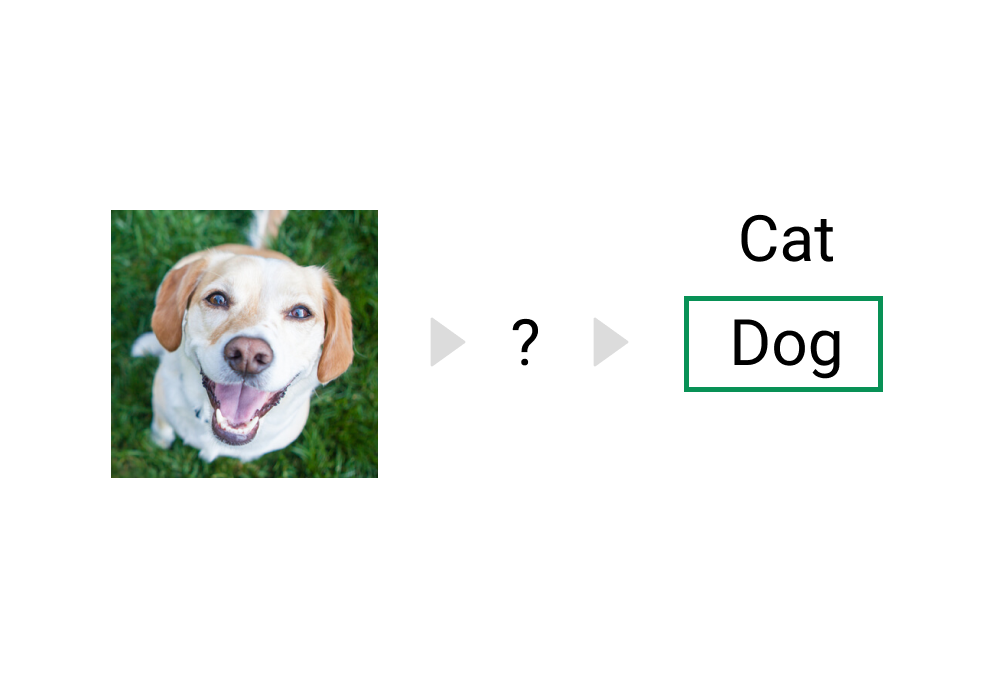# Image processing, part 2: How do image classifiers work?###### Thilo HuellmannImage classifiers are fascinating: They take an image as an input and output either a class or a probability that the image is a particular class. They can be found at the core of everything, from Facebook’s photo tagging to self-driving cars. But how do they work?

This article will give you a comprehensive overview on the technical aspects of image classifiers. It'll explain how a computer reads an image, what a CNN is and what its different layers (convolutional, ReLU, pooling and fully connected layer) do.

If you are new to the field, we recommend you first read this article, which gives a non-technical overview of what image classifiers are and where they are used.

## What a computer sees when looking at an image

The first important thing to understand is what a computer sees when looking at an image: not actual visual content but an array of numeric pixel values.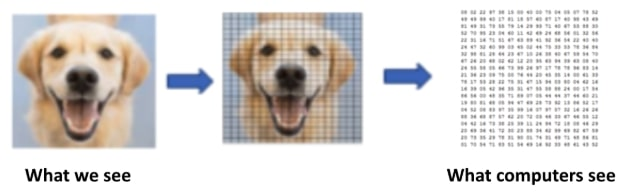How a computer's representation of an image differs from what we see.

Each pixel constitutes a single color. Each color, in turn, is made up of the three colors red, green, and blue. Each of these three colors, again, has a decimal color value. This value can range between 0 to 255, with 0 being completely black and 255 completely white. Hence, each color pixel can be described by three numbers, the so-called RGB decimal values. A pixel from the dog’s light brown fur, for instance, translates to rgb(255,248,220). These numbers are the only inputs available to the computer.

What we want the image classifier to do now is the following: take the array of numbers and output the probability of the image being a certain class (e.g. 90% for dog, 10% for cat). But how is this going to happen?

When we humans see and classify something, our brain makes sense of it by labeling, predicting, and recognizing specific patterns. Similarly, image classifiers rely on brain-like structures: so-called convolutional neural networks (CNNs).

## What is a CNN?

CNNs are a type of neural network. Neural networks try to mimic the human brain and its learning process. Like a brain takes the input, processes it and generates some output, so does the neural network by passing it through different layers. Below is a skeleton of what a neural network looks like:

The leftmost layer is called the input layer, the rightmost layer the output layer. The middle layers are called hidden layers because their values aren't observable in the training set - here’s where the magic happens. The more hidden layers a network has between the input and output layer, the deeper it is.

The great thing about neural networks is that they learn over time if their predictions are accurate - if not, they learn from their errors and update the parameters of the network. Relying on such self-learning deep networks, image classifiers belong to the field of deep learning. In case you want to dive deeper into the world of deep learning, we recommend you to check out this article.

But what makes CNNs a special form of neural networks? The answer: Their specific types and architecture of hidden layers, namely

• Convolutional layers
• ReLU layers
• Pooling layers
• Fully connected layer (the final layer)

Each layer type can occur several times. The order of the layers is not fixed but follows some rules: The network starts with a convolutional layer and ends with a fully connected layer. The fully connected layer is preceded by a final pooling layer. Therefore, an exemplary CNN could look something like this:

Input ->Convolution ->ReLU ->Convolution ->ReLU ->Pooling ->

ReLU ->Convolution ->ReLU ->Pooling ->Fully Connected -> Output

Don’t worry if you don’t yet understand what each layer does, we come to that now.

## How an image classifier works - step by step

An image classifier takes the numerical pixel values of an image, passes it through its CNN and gets a final output. As explained earlier, this output can be a single class or a probability of classes that best describes the image. The process looks like this:

Inside the hidden layers of the CNN is where the “magic” happens. On the journey from inputting a picture to outputting a class, each CNN layer type performs a specific task.

### 1. Convolution layer

Convolution layers are the major building blocks in image classifiers. The mathematical term convolution refers to the combination of two functions (f and g) that produce a third function (z). The convolutional layer takes an input, applies a filter and outputs a feature map. The feature map (z) is a combination of input and filter (f and g), hence the name convolution layer.

The objective of convolution is to extract the features of an image. A feature is a specific characteristic of the original image, such as points, edges, or the shape of the dog’s nose. Similar to the image being processed as numerics, a feature translates into a box of numeric pixel values. This matrix serves as a feature detector. Imagine you want to detect a vertical line. A simplified feature detector would look like this: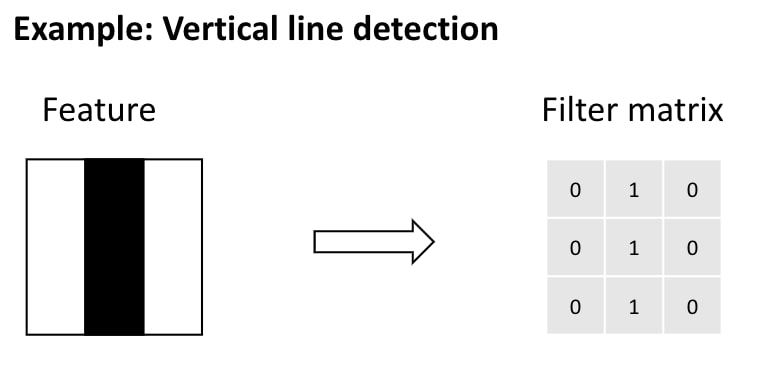A filter matrix for the detection of a vertical line is created

To scan the image, this filter matrix moves across the image, pixel block by pixel block. For each subregion, a value is calculated based on how good of a fit there is between filter and image.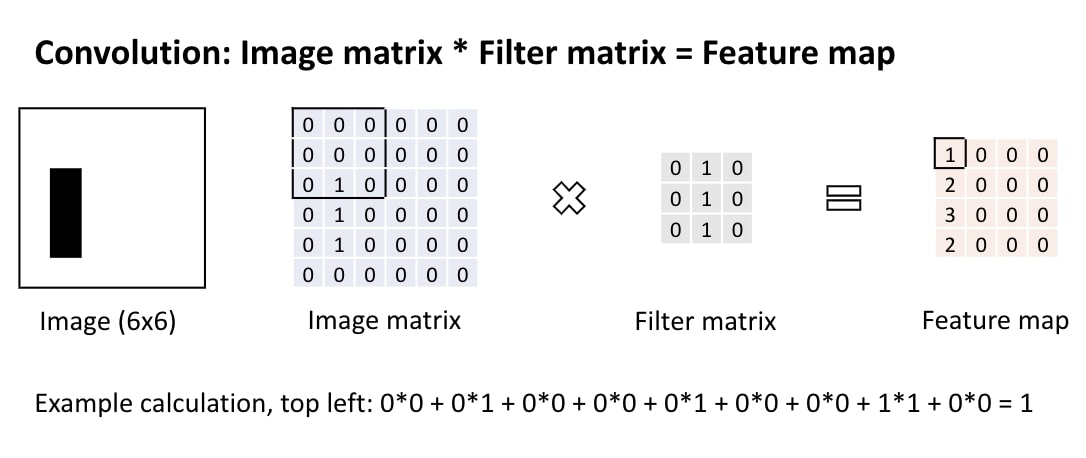Convolution: An image gets translated into a feature map by multiplying the image matrix with the filter matrix

The calculation is a simple multiplication of the two matrices. Therefore, the better the match the higher the resulting value in the feature map.

### 2. ReLU layer

ReLU is short for rectified linear unit and a so-called activation function. The main goal of using an activation function is to add non-linearity to the computation. A ReLU layer takes **the feature map (i.e. the output of the convolution layer) and rectifies any negative values to zero. Positive numbers stay the same.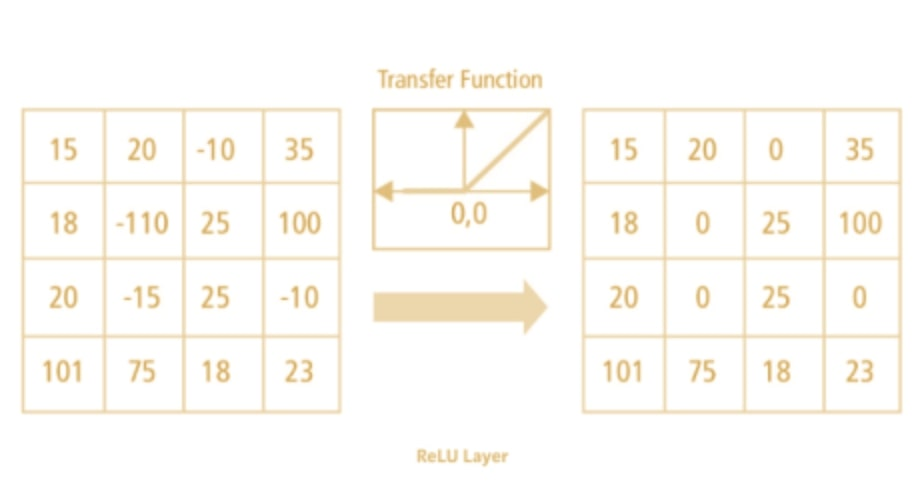The ReLU layer rectifies all negative values to zero. (Credits: Raghav Prabhu)

Non-linearity means that the slope isn’t constant. The ReLU layer is non-linear as the function’s slope is either 0 (for x < 0) or 1 for (x > 0).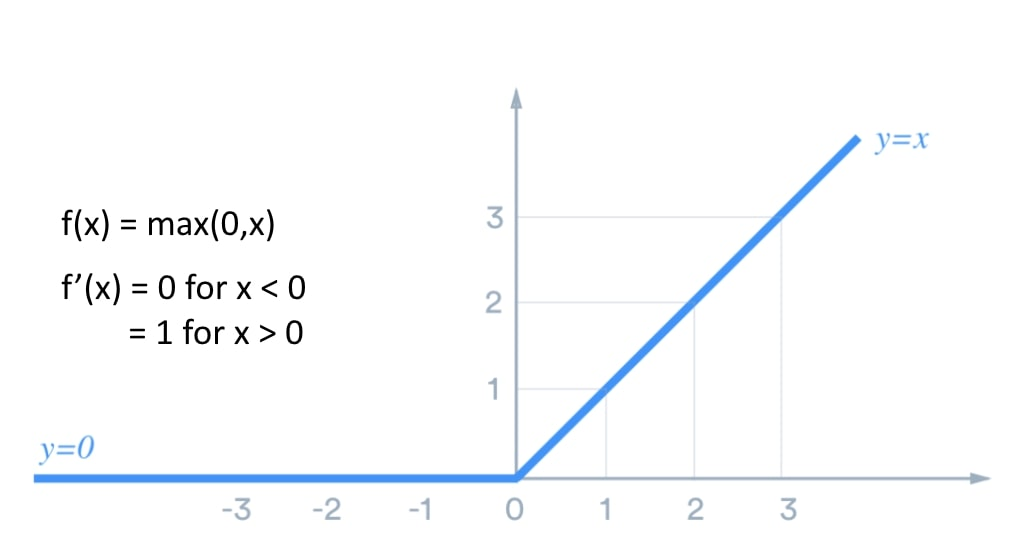The slope of the activation function is 0 for negative values and has a slope of 1 for all positive values.

Note: Image classifiers not only work with ReLU but also with other non-linear activation functions, such as tanh or sigmoid. However, ReLU is more efficient performance-wise and therefore the preferred option for most software engineers.

### 3. Pooling layer

In the process of pooling, a filter runs over the input matrix and assigns a single value per subregion to a new output matrix. The main objective of pooling is to downsize an image. This will increase the computational speed of your image classifier. The most common pooling approach is max pooling.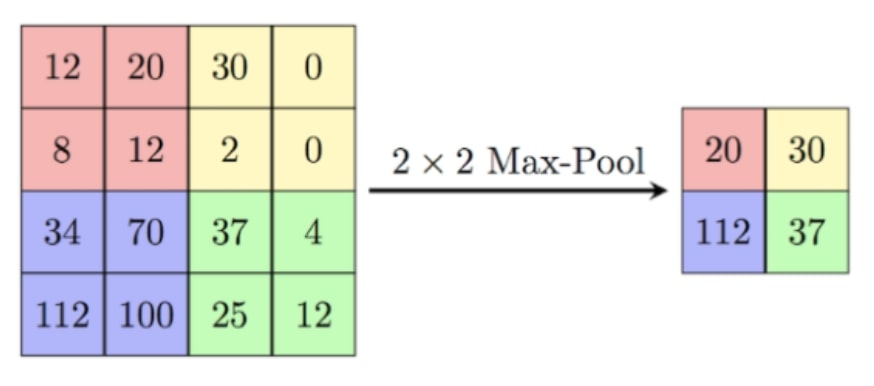In max pooling, the filter outputs the maximum value of a specific subregion of the input. (Credits: Raghav Prabhu)

In the visualization, a 2x2 filter runs over a 4x4 input matrix. The filter takes the maximum value (“max” pooling) of a specific position and assigns it as 1x1 value to the output matrix. For instance, in the four fields of the top left the highest value is 20. This number is then assigned as a single field to the output matrix. From four fields to one - that’s a data reduction of 75%!

The filter then moves on to the next position, usually without overlapping. In the resulting output matrix, each value corresponds to the maximum value of the associated subregion.

### 4. Fully connected layer

The fully connected layer concludes a CNN. Taking the output of the last pooling layer as an input, it aggregates all information and generates the final classification.

At this stage, each value of the input vector represents the likelihood of a feature belonging to a specific class. For instance, one value might suggest the paw belonging to a dog at a 90% likelihood. Another value might correspond to the nose. The fully connected layer takes all the information, applies a weighting and outputs a final classification.

Now the computer is ready to do the work for you.

Let’s summarize what we have learned about how image classifiers work:

• Image classifiers rely on convolutional neural networks (CNNs) to process an image. CNNs are a special form of neural networks with a specific architecture of layers.
• The four types of CNN layers are convolutional layer, ReLU layer, pooling layer, and fully connected layer. An image classifier passes an image through these layers to generate a classification.
• The convolutional layer extracts the features of an image by scanning through the image with filters.
• The ReLU layer rectifies al negative values to zero. Its objective is to add non-linearity to the model.
• Pooling layers are applied to downsize an image and increase computational speed. The most common approach is max pooling, which takes the maximum value of each subregion.
• The fully connected layer concludes the CNN. It aggregates and weights all information and generates the final classification.

Computerphile has released two great videos on Convolutional Neural Networks. Worth watching if you want to get a visual understanding.

#### More from our Blog#### Mapping the no-code AI landscape

A while ago we realized how powerful no-code AI truly is – and we thought it would be a good idea to map out the players on the field.#### Remote work: Not a trend but the new normal

Working from home does not equal working remotely, even if they overlap significantly and pose similar challenges – remote work is also a mindset.